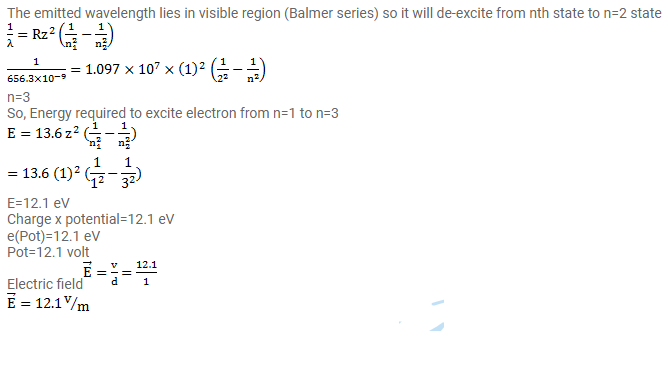# Electrons are emitted from an electron gun at almost zero velocity and

Question:

Electrons are emitted from an electron gun at almost zero velocity and are accelerated by an electric field $E$ through a distance of $1.0 \mathrm{~m}$. The electrons are now scattered by an atomic hydrogen sample in ground state. What should be the minimum value of E so that red light of wavelength $656.3 \mathrm{~nm}$ may be emitted by the hydrogen?

Solution: